What is the power factor of an PV or wind power inverter?

## Overview

Inverters are generally designed to generate power at unity power factor, particularly at full power. The actual requirements vary, but one example is:

`The power factor must be greater than 0.90 for generated power greater than or equal to 50% of full power.`

Unfortunately, older inverter designs have poor power factors when operating at low power levels. Filter capacitors on the inverter output, which are used to filter the high-frequency switching noise, can cause low power factors. When the generated power is high, the capacitive load becomes a small fraction of the total and has little effect on the power factor, but at low power levels, this capacitive load can result in power factors as low as 0.5.

The power factor of newer designs is typically adjustable from –0.80 to 0.85 or higher.

## Measuring or Estimating Power Factor

You can use our WattNode meters (those that report power factor) to directly measure the inverter power factor. Alternatively, you can estimate the power factor of an inverter. This estimate may not work for all inverters, depending on their control logic and output filter circuitry. The following equations disregard the real power consumption of an inverter when it is idle, which may range from a fraction of a watt to a few watts for larger inverters. Generally, the reactive power due to the filter capacitors ranges from tens to hundreds of VARs, so the small idle power is negligible by comparison when estimating the power factor.

General Equations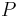$P$ real power (watts)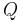$Q$ reactive power (VARs)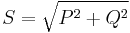$S = sqrt{P^2 + Q^2}$ apparent power (VAs)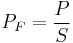$P_F = frac{P}{S}$ power factor

Inverter Power Factor Equations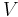$V$ nominal inverter voltage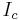$I_c$ current due to inverter filter capacitors, measure when inverter is not generating any power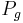$P_g$ real power (watts), inverter generated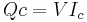$Qc = V I_c$ reactive power (VARs) due to filter capacitors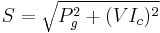$S = sqrt{P_g^2 + (V I_c)^2}$ apparent power (VAs)$P_F = frac{P_g}{sqrt{P_g^2 + (V I_c)^2}}$ power factor

In real life, the power factor will generally be higher than 0.9 unless the generated power is low, probably less than 20% of full-scale. So you could simplify and assume near unity power factor when generating and near zero power factor the rest of the time. But if you have an oversized inverter running in the 10-20% of full-scale range, you may measure power factor values far lower than 0.9, perhaps 0.5.